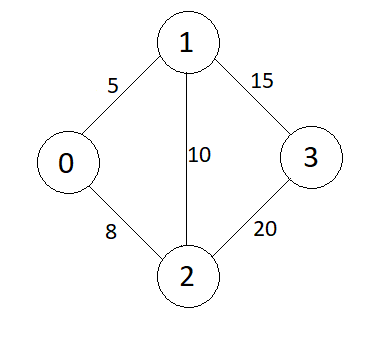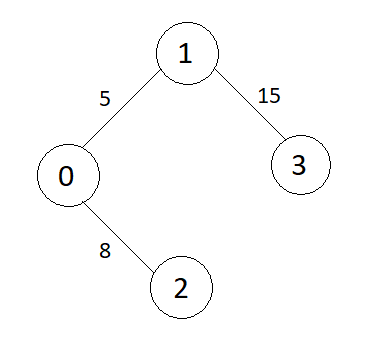New update is available. Click here to update.

# Prim's MST

Last Updated: 17 Jan, 2021
Difficulty: Moderate

## PROBLEM STATEMENT

#### You are given an undirected connected weighted graph having ‘N’ nodes numbered from 1 to 'N'. A matrix ‘E’ of size M x 2 is given which represents the ‘M’ edges such that there is an edge directed from node E[i] to node E[i]. You are supposed to return the minimum spanning tree where you need to return weight for each edge in the MST.

##### For example :``````The MST (Minimum Spanning Tree) for the above graph is:
``````##### Input Format :
``````The first line of input contains an integer 'T' representing the number of the test case. Then the test cases are as follows.

The first line of each test case argument given is an integer ‘N’ representing the number of nodes in the graph.

The second line of each test case contains a given integer ‘M’ representing the number of edges.

The next ‘M’ lines in each test case contain a matrix ‘E’ of size M x 2 which represents the ‘M’ edges such that there is an edge directed from node E[i] to node E[i].
``````
##### Output Format :
``````For each test case, print the minimum spanning tree in the form of edges and their weights which are included in the MST.
``````
##### Note :
``````You do not need to print anything; It has already been taken care of.
``````
##### Constraints :
``````1 ≤ T ≤ 5
2 <= N <= 100
1 <= M <= min(1000, N(N - 1) / 2)
1 <= E[i], E[i] <= N

Time Limit: 1 sec
``````